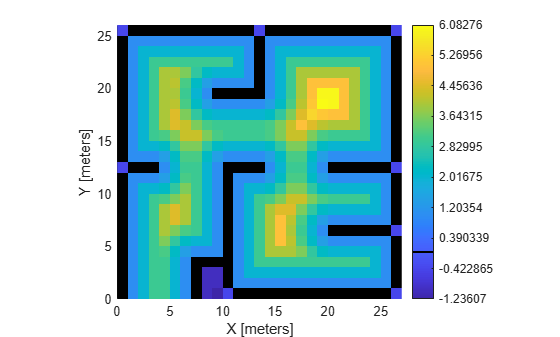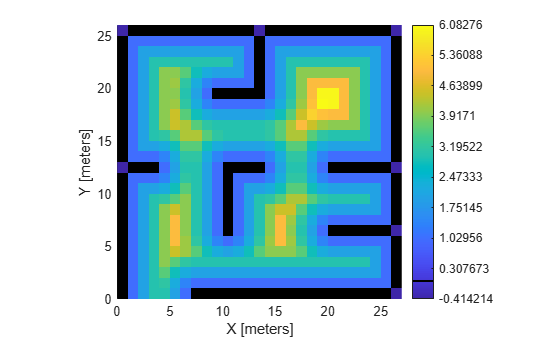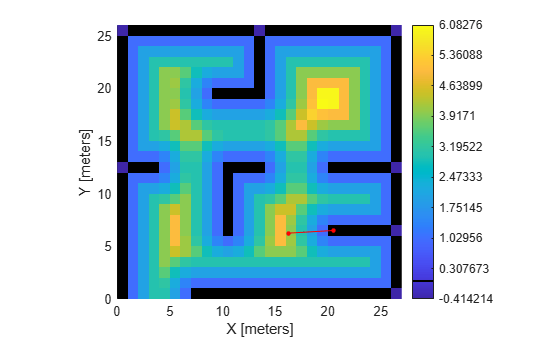# distance

Get distance at locations

Since R2023a

## Syntax

``dist = distance(map)``
``dist = distance(map,location)``
``dist = distance(map,location,frame)``
``[dist,isValid] = distance(map,location,___)``
``dist = distance(map,cornerLocation,mapSize)``
``dist = distance(map,cornerLocation,mapSize,frame)``

## Description

````dist = distance(map)` returns the signed distances `dist` to the closest obstacle for all cells in the map `map`.```
````dist = distance(map,location)` returns the signed distances to the closest boundaries for the specified xy-locations `location` in world coordinates. NoteNote that the `distance` function determines the distance using the interpolation method specified in `InterpolationMethod` property of `map`. ```
````dist = distance(map,location,frame)` returns distances to the closest boundaries for the specified locations `location` in the coordinate frame `frame`.```

example

````[dist,isValid] = distance(map,location,___)` returns `isValid`, indicating which of the specified locations `location` are within the map bounds `isValid`.```
````dist = distance(map,cornerLocation,mapSize)` returns the distances to the closest boundaries for a subregion of the map layer, `map`. The subregion starts in the corner location `cornerLocation` in the world coordinate frame with a given map size `mapSize`.```
````dist = distance(map,cornerLocation,mapSize,frame)` returns the distances to the closest boundaries for a subregion of the map layer, `map`. The subregion starts in the corner location `cornerLocation` in the coordinate frame `frame`. NoteWhen specifying a corner location and map size, `distance` determines the distances between cell centers within the rectangular query region. ```

## Examples

collapse all

Load the `exampleMaps` MAT file.

`load exampleMaps.mat`

Create a signed distance map using the `simpleMap` data.

```sdm = signedDistanceMap(simpleMap,InterpolationMethod="none"); show(sdm,BoundaryColor=[0 0 0],Colorbar="on");``````setMapData(sdm,[7 1],zeros(5,5)) getMapData(sdm,[9 2])```
```ans = logical 0 ```
`show(sdm,BoundaryColor=[0 0 0],Colorbar="on");`Find the closest boundary to the coordinate, `[16.25 6.25]` and calculate the distance to the nearest boundary.

```coord = [16.25 6.25]; boundary = closestBoundary(sdm,coord,"world")```
```boundary = boundary(:,:,1) = 20.5000 boundary(:,:,2) = 6.5000 ```
`dist = distance(sdm,coord)`
```dist = 4 ```

Plot the line between the queried point and the closest obstacle cell center

```hold on plot([coord(1) boundary(:,:,1)],[coord(2) boundary(:,:,2)],"-r",Marker=".",MarkerSize=10)```Note that even though distance from the queried point appears greater than `4` when plotted, the distance function calculates the distance from the nearest cell center of the queried point.

## Input Arguments

collapse all

Signed distance map, specified as a `signedDistanceMap` object.

World or local coordinates, or grid locations, specified as an N-by-2 matrix. N is the number of locations. The format of the rows depends on the value of the `frame` argument:

• `"world"` — [x y] coordinates in the world frame.

• `"local"` — [x y] coordinates in the local frame.

• `"grid"` — [row column] location in the grid frame.

Data Types: `double`

Location of output matrix, specified as a two-element vector of coordinates in the form, [x y]. The location is in world or local coordinates if the `frame` argument is set to `"world"` and `"local"`, respectively. When `frame` is `"grid"`, `cornerLocation` is the top-left corner of the world frame.

Data Types: `double`

Subregion map size, specified as a two-element vector [x y] in world or local coordinates. Location is in world or local coordinates if the `frame` argument is set to `"world"` and `"local"`, respectively. When `frame` is `"grid"`, `cornerLocation` is the top-left corner of the world frame.

Data Types: `double`

Coordinate frame, specified as one of these options:

• `"world"` — Specify coordinates in the world frame.

• `"local"` — Specify coordinates in the local frame.

• `"grid"` — Specify grid locations in the grid frame.

Data Types: `char` | `string`

## Output Arguments

collapse all

Distances to closest obstacles, returned as an L-element column vector for location queries and an M-by-N-by-2 array for subregion queries. L is the number of locations queried. M and N are the dimensions of the map or specified map subregion.

If `frame` is either `"world"` or `"local"`, then `distance` returns distances in Cartesian space.

If `frame` is `"grid"`, then `distance` returns distances in grid space.

Points in boundary, returned as an L-element vector, where L is the total number of queried locations. Each element is a `1` (`true`) if the point is within the boundaries, and `0` (`false`) if the point is outside boundaries.

This output is only returned for point queries.

## Version History

Introduced in R2023a